# Primary 6 PSLE Practice #1

Time elapsed:
Q1. Which pair of lines is parallel?     [1 mark]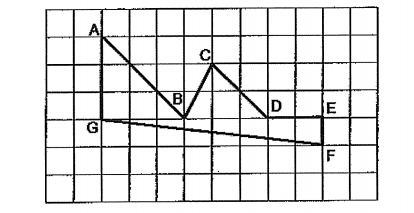Q2. The figure below is made up of a rectangle and 3 identical circles. Find the area of the shaded part. Leave your answer in terms of π.     [2 marks]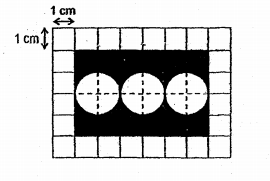Q3. The distance between the first lamp-post and the fifth lamp-post is 20 m. If the lamp-posts are spaced out equally, what is the distance between the first and the third lamp-post?     [1 mark]
Q4. In the diagram below, ABCD is a parallelogram and PQRS is a rhombus. PB = BN, ∠APS = 40° and ∠PQR = 86°. Find ∠ADC.     [2 marks]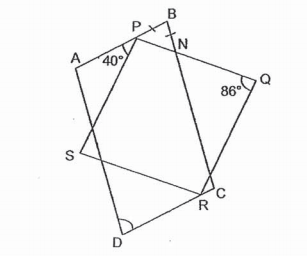Q5. Express 56 ÷ 2 in its simplest form.     [1 mark]
Q6. Express 6 2500 as a decimal.     [1 mark]
Q7. Simplify the expression 9y + 7 - 5y + 3.     [1 mark]
Q8. Shawn puts 40 apples into a carton. There are 24 red ones and the rest are green. Find the ratio of the number of green apples to that of the total number of red and green apples.     [2 marks]
Q9. The figure below shows an isosceles triangle WXY. ∠YWX = 58°. Find ∠WXY.     [1 mark]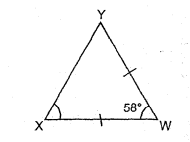Q10. A car left village A and travelled at an average speed of 70 km/h towards Town P. A coach left village B and travelled at an average speed of 50 km/h toward Town Q. Village A and Village B are 10 km apart. How far apart are the two vehicles one hour after the drivers have started their journeys?     [1 mark]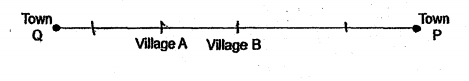Q11. 14 of a pole is painted white and 12 of the reminder is painted red. What fractions of the pole is not painted?     [2 marks]
Q12. Zoe took a flight from 06 45 to 16 00. How long was the flight?     [1 mark]
Q13. Zoe had 9 kg of flour. She used 13 of it to bake a cake. After that, she used 12 kg of the flour to bake some cookies. How much flour had she left?     [2 marks]
Q14. 120 girls and 80 boys went to a camp. What percentage of the children were girls?     [1 mark]
Q15. Nathaniel weighs 3x kg. Alex is twice as heavy as Nathaniel. Kingston is 2x kg lighter than Alex. What is their total weight?     [2 marks]
Q16. Each figure below is made up of nine squares. Four squares in each figure are shaded. Which one of the following figures are symmetric?     [1 mark]
Q17. Beatrice threads circular beads on a string 91 cm long in a straight line. The beads follow a repeated pattern without gaps between them as shown below. Each bead has a radius of 0.5 cm and is black, white, grey or striped. The first bead and the last bead are positioned 15 cm from the respective ends of the string. What is the colour of the last bead?     [2 marks]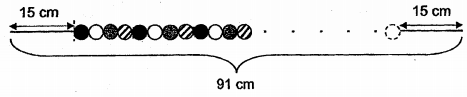Q18. A piece of string is cut into two pieces, which are used to from two squares. The sum of the areas of the two squares is 45 cm2. The length of the smaller square is 3 cm. What is the length of the bigger square?     [2 marks]
Q19. Express 6 912 as a decimal.     [1 mark]
Q20. Yvette is y years old this year. Her brother is twice as old as her. How old was her brother 2 years ago?     [1 mark]